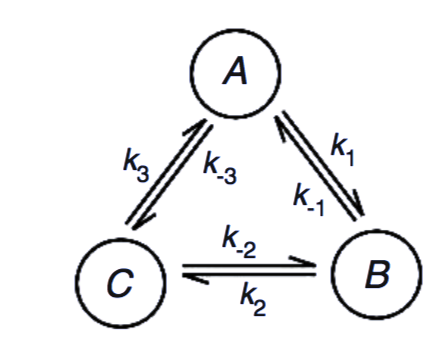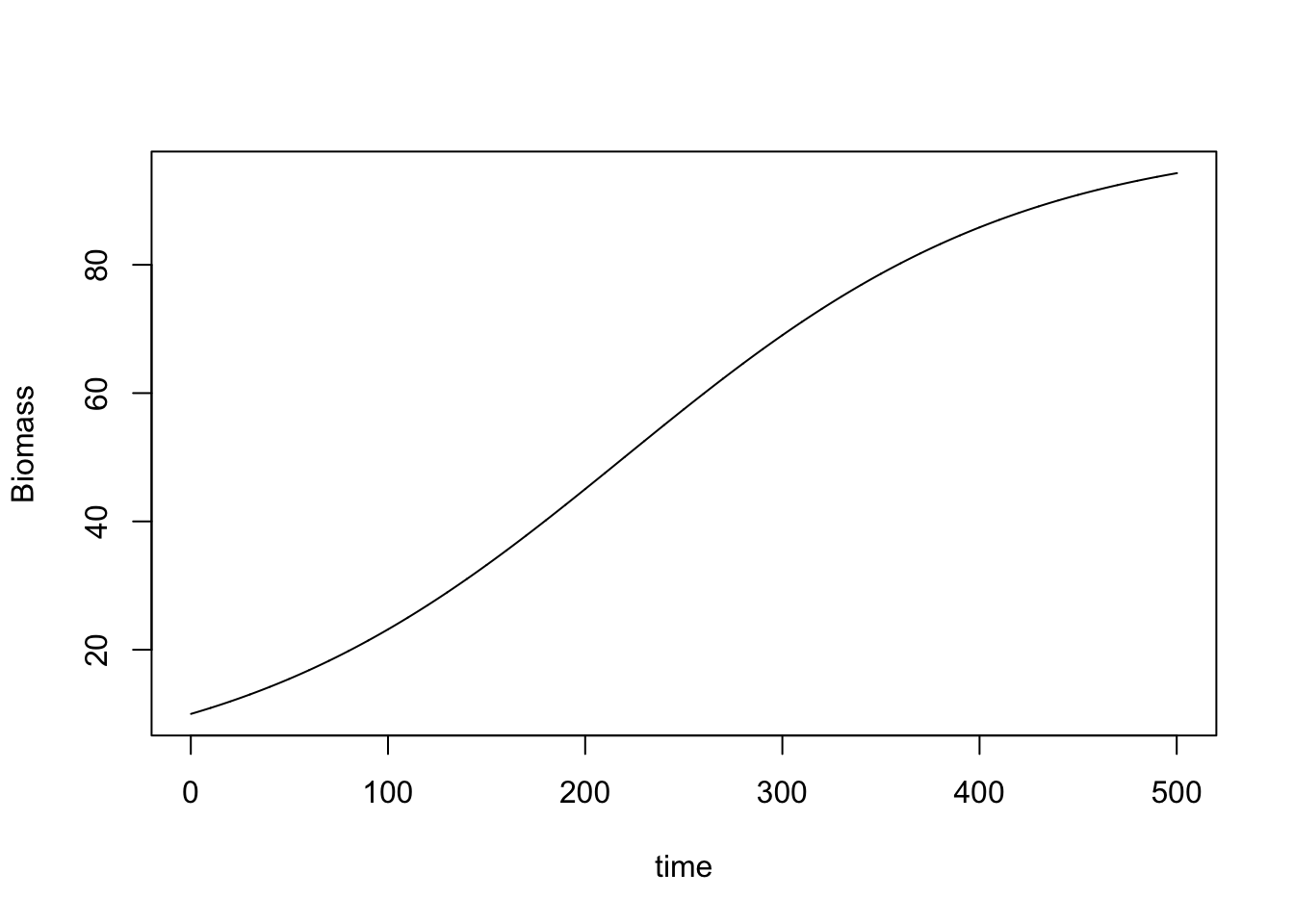## Examples of a Linear equation

x + y + z = 6
2y + 5z = −4
2x + 5y − z = 27

The matrix form of the equation is :
$A u = v$

where,
$A=\left(\begin{array}{ccc} 1 &1 & 1\\ 0&2&5\\ 2&5&1\\ \end{array}\right)\\ u=\left(\begin{array}{c} x\\ y\\ z\\ \end{array}\right)\\ v=\left(\begin{array}{c} x\\ y\\ z\\ \end{array}\right)$

The solution of the above equation in matrix form is given by,

$u =A^{-1}v$

## Problem:

How to tackle situtations when det|A|=0. Will inverse of A exist?

## Inverting a matrix in R

library(matlib)
cat("A=")
## A=
A <- matrix( c(1, 1, 1,0,2,5,2,5,-1),nrow=3, byrow=TRUE)
(A) 
##      [,1] [,2] [,3]
## [1,]    1    1    1
## [2,]    0    2    5
## [3,]    2    5   -1
cat("inverse A=")
## inverse A=
(AI<-inv(A))
##            [,1]       [,2]       [,3]
## [1,]  1.2857143 -0.2857143 -0.1428571
## [2,] -0.4761905  0.1428571  0.2380952
## [3,]  0.1904762  0.1428571 -0.0952381
vec=matrix(c(6,-4,27),nrow=3, byrow=TRUE)
(vec)
##      [,1]
## [1,]    6
## [2,]   -4
## [3,]   27
u<-AI%*%vec
x<-u
y<-u
z<-u

s<-sprintf("x=%6.3f ,y=%6.3f ,z=%6.3f",x,y,z)
cat(s)
## x= 5.000 ,y= 3.000 ,z=-2.000

## Transpose of a matrix

cat("Transpose of A=")
## Transpose of A=
(A.t<-t(A))
##      [,1] [,2] [,3]
## [1,]    1    0    2
## [2,]    1    2    5
## [3,]    1    5   -1

## Product of two matrices

cat("inverse A multiplied by A=")
## inverse A multiplied by A=
B<-AI%*%A

(round(B))
##      [,1] [,2] [,3]
## [1,]    1    0    0
## [2,]    0    1    0
## [3,]    0    0    1

## Matrix in Chemistry

Let us now look at the following reactionWe can write the reaction velocity v $v=\left(\begin{array}{c} v_1\\ v_2\\ v_3\\ \end{array}\right)$

concentration by : $u=\left(\begin{array}{c} A\\ B\\ C\\ \end{array}\right)$

The following rate relation follows:

1. $du/dt = \Gamma v,v= K u\\$ where, $K=\left(\begin{array}{ccc} k_1 & -k_1 & 0\\ 0 & k_2 & -k_2\\ -k_1 & 0 & k_3\\ \end{array}\right)\\$ and, $\Gamma=\left(\begin{array}{ccc} -1 &0& 1\\ 1&-1&0\\ 0&1&-1\\ \end{array}\right)\\$

As the conservation equation A+B+C= constant , holds good i.e.,

1. $d/dt (\Sigma u) =d/dt(A+B+C)=0$ theer are only two independent variables. We can write , $\partial C =-(\partial A +\partial B )$ What follows is a dimensionally reduced scenario,
2. $\frac{du}{dt}= \alpha u, \alpha = \Gamma K$

## Solution to differential equation

As the equation (2) implies reduced dimensionality the 3X3 matrix B in equation (3) has zero determinant.

### Excercise

Reduce $$\alpha$$ to a 2D matrix $$\beta$$ and write down its explicit form.

### Solution

1. $d\psi /dt = \beta \psi, \psi=(\partial a,\partial B)$

where,
$\beta=\left(\begin{array}{cc} -k_{-1}-k_{-3}-k_3 & k_{-1} -k_3 \\ k_1- k_{-2} & k_{-1}-k_2-k_{-2}\\ \end{array}\right)\\$

### Solving using Eigen value

Plz solve . Show that the eigen value of the above matrix is always real and negative (the system will reach equilibrium without ocsillation)provided the following is true : $k_1.k_2.k_3=k_{-1}.k_{-2}.k_{-3}$ In constrast show that pure osciilation will occur (eigen value of the rate matrix will be purely imaginary ) in case of classical SHM.

### Euler Algoritm Pseudo Code

input t0 and y0.
input step size, h and the number of steps, n.
for j from 1 to n do
m = f (t0, y0)
y1 = y0 + h*m
t1 = t0 + h
Print t1 and y1
t0 = t1
y0 = y1
end

### Using Euler algoritm for the logistic rate equation

Let us assume that we deal with Logistic Equation

$\frac{dx}{dt}= rx (1-\frac{x}{K})$ This equation can be used for studying growth dynamics of different biomasses ( plant,bacteria,fungi,fish). (See Gordon Model for open access fishery).

x=10.0
r=0.01
K=100.0
n=5000
tau=0.1
tmax=5000.0
t=0.1
s<-c(x)
tt<-c(t)
for (i in 1:n){
x<-x+tau*r*x*(1-x/K)
s<-c(s,x)
x
t<-t+tau
tt<-c(tt,t)
}
plot(tt,s,type='l',xlab="time",ylab="Biomass")